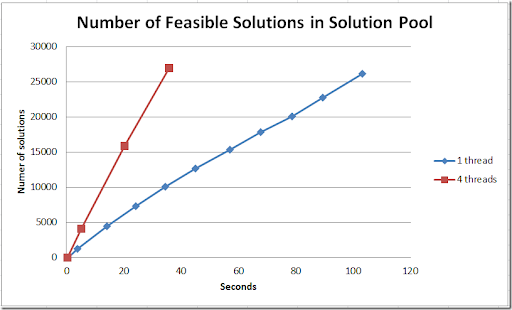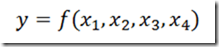## Thursday, August 29, 2013

### Pindyck

Robert Pindyck in “CLIMATE CHANGE POLICY: WHAT DO THE MODELS TELL US?”:

ABSTRACT: Very little. ….

One of the problems with these long range models is the use a discount rate. This rate can have large effects on the model results. This is true in many models with long planning horizons. One should be aware of this, e.g. by running different scenarios with different discount rates to see what the impact is on the solutions.

Listen also to: http://www.econtalk.org/archives/2013/08/pindyck_on_clim.html.

## Tuesday, August 27, 2013

### Cplex solution pool

I am working on a fairly complex but small MIP model, where we want to find a large number of non-dominated, feasible solutions (Pareto solutions). In one of the models we have developed for this, we use Cplex to quickly give us all solutions in a certain neighborhood using the solution pool facilities (we filter out dominated solutions later on). As the models are fairly small, it was not immediately obvious if more cores or cpus would help. It turns out it really does:### 2D Interpolation With SOS2 variables

Using SOS2 variables to implement a 1D interpolation scheme is fairly easy (see: http://yetanothermathprogrammingconsultant.blogspot.com/2009/06/gams-piecewise-linear-functions-with.html). However, a 2D problem is already much more difficult. Here is an example taken from the Lindo web site:

 \$ontext   2D Interpolation with SOS2 variables   See: http://www.lindo.com/cgi-bin/modelf.cgi?Piecelin2Dsos3.txt;LINGO \$offtext \$set  n1 3 \$set  n2 3 \$eval n3 (%n1%+%n2%-1) sets    i /i1*i%n1%/    j /j1*j%n2%/    k /k1*k%n3%/ ; table data(i,j,*)            x   y   f i1.j1    195 1800  20 i1.j2    217 1900  26 i1.j3    240 2000  30 i2.j1    195 3500  52 i2.j2    217 3600  61 i2.j3    240 4100  78 i3.j1    195 5100  69 i3.j2    217 5200  80 i3.j3    240 5600  93 ; parameters    xv(i,j)    yv(i,j)    fv(i,j) ; xv(i,j) = data(i,j,'x'); yv(i,j) = data(i,j,'y'); fv(i,j) = data(i,j,'f'); sos2 variables   wx(i)   wy(j)   wd(k) ; positive variables   WGT(i,j)   xa   ya   fa ; variables z; equations    xconvex    yconvex    dconvex    ewx    ewy    ewd    compx    compy    compfv    obj ; xconvex.. sum(i, wx(i)) =e= 1; yconvex.. sum(j, wy(j)) =e= 1; dconvex.. sum(k, wd(k)) =e= 1; ewx(i)..  wx(i) =e= sum(j, wgt(i,j)); ewy(j)..  wy(j) =e= sum(i, wgt(i,j)); ewd(k)..  wd(k) =e= sum((i,j)\$(ord(i)+ord(j)-1=ord(k)), wgt(i,j)); compx.. xa =e= sum((i,j), xv(i,j)*wgt(i,j)); compy.. ya =e= sum((i,j), yv(i,j)*wgt(i,j)); compfv.. fa =e= sum((i,j), fv(i,j)*wgt(i,j)); obj..  z =e= YA + 15*XA; fa.lo = 67; xa.lo = 227; xa.up = 229; model m /all/; solve m minimizing z using mip;

### 4D Interpolation

For a engineering design application we were offered a 4D lookup table that predictsOf course rather than “lookup” it is often better to do interpolation, so values in between are accepted. This still looks pretty bad. Our model was a MIP and using SOS variables we can easily do 1D and with some effort do 2D. For more dimensions, things are really difficult.

Luckily it turned out that we know the values of x1,x2 and x3 before we start the optimization model. I.e. we can do a 3D Interpolation in GAMS, followed by a 1D interpolation with SOS2 variables in Cplex.

For the 3D interpolation we used a simple IDW (Inverse Distance Weighting) scheme (explained in: http://en.wikipedia.org/wiki/Inverse_distance_weighting). We do this a number of times so we can estimate a good set of control points for the remaining 1D interpolation. This was done by a piecewise linear function, as explained here: http://yetanothermathprogrammingconsultant.blogspot.com/2009/06/gams-piecewise-linear-functions-with.html.